# Application to linear models

### Application to linear models

#### Lessons

In this section, we will apply least square problems to economics.

Instead of finding the least squares solution of $Ax=b$, we will be finding it for $X\beta =y$ where

$X$→ design matrix
$\beta$→ parameter vector
$y$→ observation vector

Least-Squares Line
Suppose we are given data points, and we want to find a line that best fits the data points. Let the best fit line be the linear equation
$y=\beta_0 + \beta_1 x$

And let the data points be $(x_1,y_1 ),(x_2,y_2 ),\cdots,,(x_n,y_n )$. The graph should look something like this: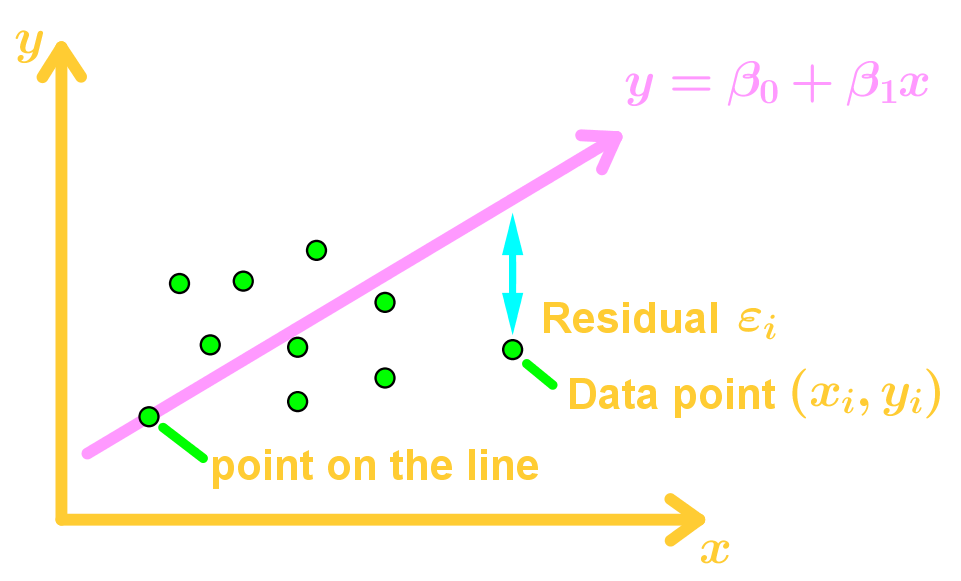Our goal is to determine the parameters $\beta_0$ and $\beta_1$. Let's say that each data point is on the line. Then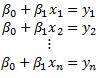This is a linear system which we can write this as: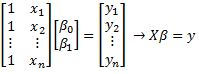Then the least squares solution to $X\beta=y$ will be $X^T X \beta =X^T y$.

General Linear Model
Since the data points are not actually on the line, then there are residual values. Those are also known as errors. So we introduce a vector called the residual vector $\epsilon$, where
$\epsilon = y - X\beta$
$y = X\beta + \epsilon$

Our goal is to minimize the length of $\epsilon$ (the error), so that $X\beta$ is approximately equal to $y$. This means we are finding a least-squares solution of $y=X\beta$ using $X^T X\beta=X^T y$.

Least-Squares of Other Curves
Let the data points be $(x_1,y_1 ),(x_2,y_2 ),\cdots,,(x_n,y_n )$ and we want to find the best fit using the function $y=\beta_0+\beta_1 x+\beta_2 x^2$, where $\beta_0,\beta_1,\beta_2$ are parameters. Technically we are using a best fit quadratic function instead of a line now.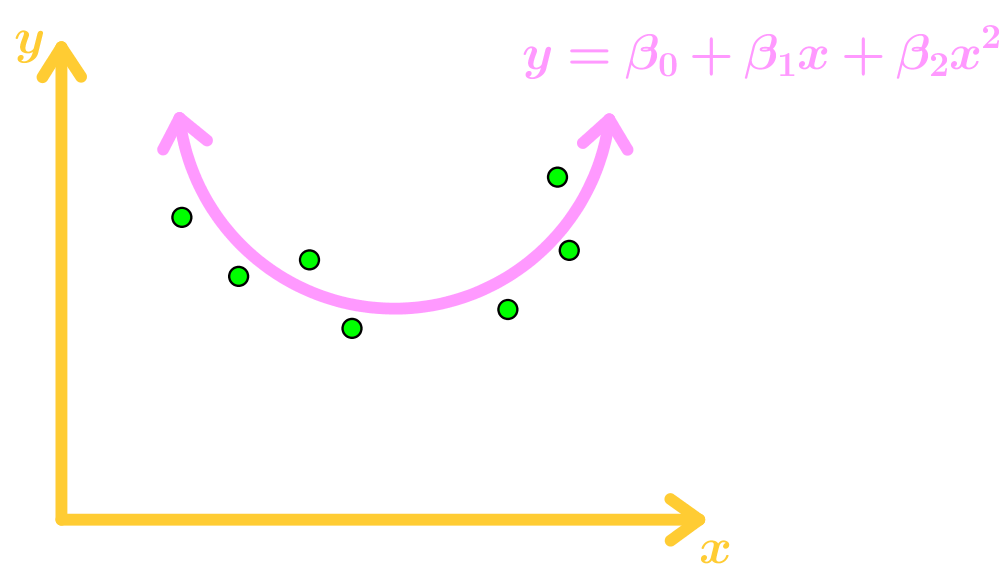Again, the data points don't actually lie on the function, so we add residue values $\epsilon_1,\epsilon_2,\cdots,\epsilon_n$ where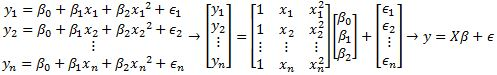Since we are minimizing the length of $\epsilon$, then we can find the least-squares solution $\beta$ using $X^T X\beta=X^T y$. This can also be applied to other functions.

Multiple Regression
Let the data points be $(u_1,v_1,y_1 ),(u_2,v_2,y_2 ),\cdots,,(u_n,v_n,y_n )$ and we want to use the best fit function $y=\beta_0+\beta_1 u+\beta_2 v$, where $\beta_0,\beta_1,\beta_2$ are parameters.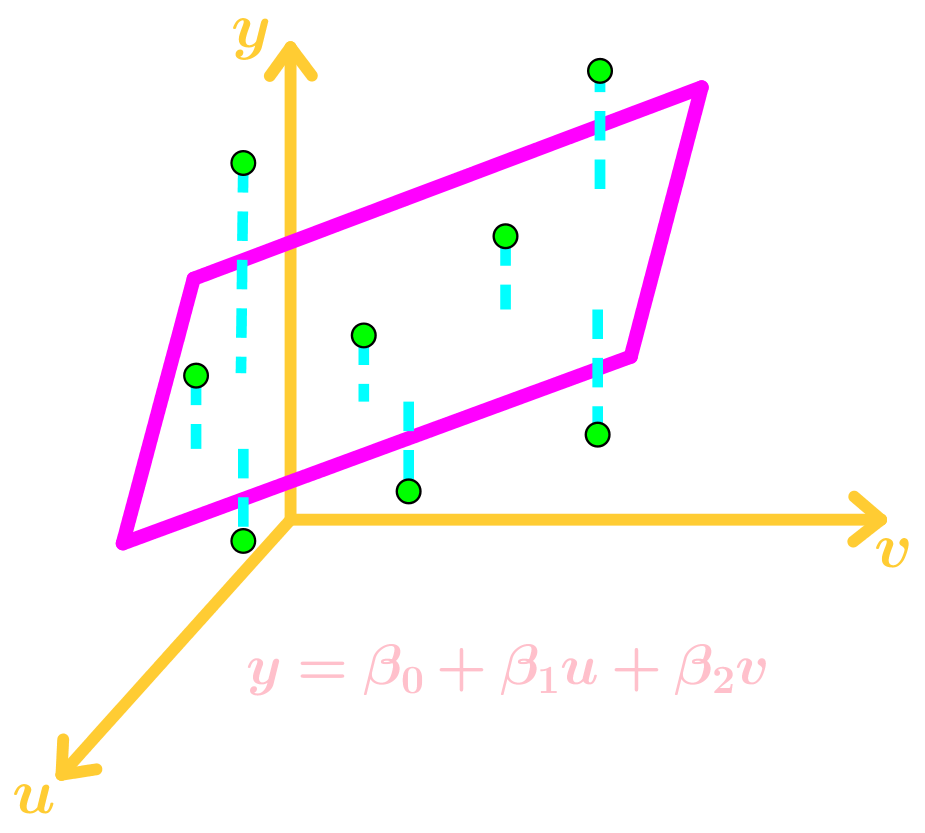Again, the data points don't actually lie on the function, so we add residue values $\epsilon_1,\epsilon_2,\cdots,\epsilon_n$ where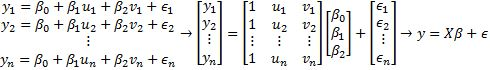Since we are minimizing the length of $\epsilon$, then we can find the least-squares solution $\beta$ using $X^T X\beta=X^T y$. This can also be applied to other multi-variable functions.
• Introduction
Applications to Linear Models Overview:
a)
Applying Least-Squares Problem to Economics
• Go from $Ax=b$ to $X\beta=y$
$X$→ design matrix
$\beta$→ parameter vector
$y$→ observation vector

b)
Least-Squares Line
• Finding the best fit line
• Turning a system of equations into $X\beta =y$
• Using the normal equation $X^T X\beta=X^T y$
• Introduction of the residual vector

c)
Least-Squares to Other Curves
• Finding the Best Fit Curve (not a line)
• Using the normal equation $X^T X\beta=X^T y$

d)
Least-Squares to Multiple Regressions
• Multiple Regression → multivariable function
• Finding a Best Fit Plane
• Using the normal equation $X^T X\beta=X^T y$

• 1.
Finding the Least-Squares Line
Find the equation $y=\beta_0+\beta_1 x$ of the least-squares line that best fits the given data points:
$(0,1),(1,2),(2,3),(3,3)$

• 2.
Finding the Least-Squares of Other Curves
Suppose the monthly costs of a product depend on seasonal fluctuations. A curve that approximates the cost is
$y= \beta _0 + \beta _1 x+ \beta _2 x^2 + \beta_3 \cos$ ($\frac{2 \pi x}{12}$)

Suppose you want to find a better approximation in the future by evaluating the residual errors in each data point. Let's assume the errors for each data point to be $\epsilon_1,\epsilon_2,\cdots,\epsilon_n$.
Give the design matrix, parameter vector, and residual vector for the model that leads to a least-squares fit for the equation above. Assume the data are $(x_1,y_1 ),\cdots,(x_n,y_n).$

• 3.
An experiment gives the data points $(0,1) , (1,3) , (2, 4), (3, 5)$. Suppose we wish to approximate the data using the equation
$y=A+Bx^2$

First find the design matrix, observational vector, and unknown parameter vector. No need to find the residual vector. Then find the least-squares curve for the data.

• 4.
Finding the Least Squares of Multiple Regressions
When examining a local model of terrain, we examine the data points to be $(1,1, 3), (2, 2, 5),$ and $(3, 1, 3)$. Suppose we wish to approximate the data using the equation
$y=\beta_0 u+\beta_1 v$

First find the design matrix, observational vector, and unknown parameter vector. No need to find the residual vector. Then find the least-squares curve for the data.

• 5.
Proof Question Relating to Linear Models
Show that
$\lVert X \hat{\beta} \rVert^2=\beta^TX^Ty$